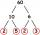# Expression of a variable from the formula + integers - math problems

1. SimplifySimplify expression - which expression is equivalent to: 3(m + 2) − 4(2m − 9)
2. Collection of stampsJano, Rado, and Fero have created a collection of stamps in a ratio of 5: 6: 9. Two of them had 429 stamps together. How many stamps did their shared collection have?
3. Four integersFnd four consecutive integers so that the product of the first two is 70 times smaller than the product of the next two.
4. ValueDetermine the value of the following exspressions: a) (23-25)·(4-5) b) (97-123):(18+8)
5. Points on circleIn the Cartesian coordinate system with the origin O is a sketched circle k /O; r=2 cm/. Write all the points that lie on a circle k and whose coordinates are integers. Write all the points that lie on the circle I / O; r=5 cm / and whose coordinates are i

We apologize, but in this category are not a lot of examples.
Do you have an interesting mathematical word problem that you can't solve it? Enter it, and we can try to solve it.

We will send a solution to your e-mail address. Solved examples are also published here. Please enter the e-mail correctly and check whether you don't have a full mailbox.

Please do not submit problems from current active competitions such as Mathematical Olympiad, correspondence seminars etc...